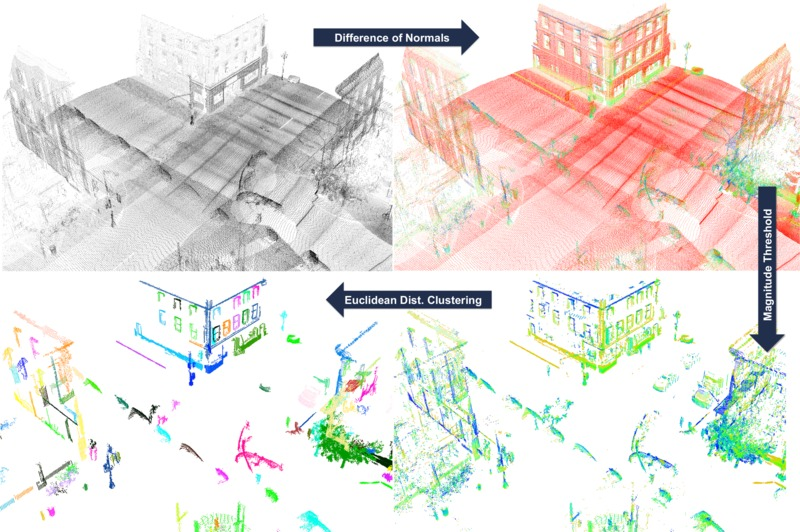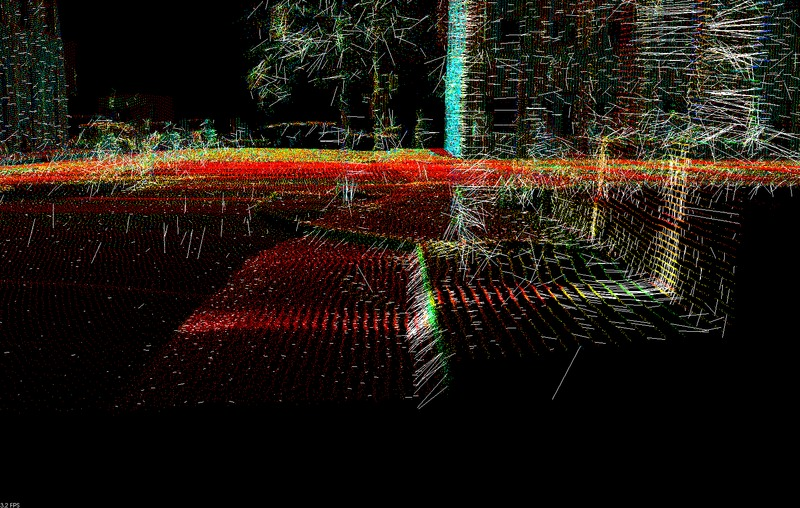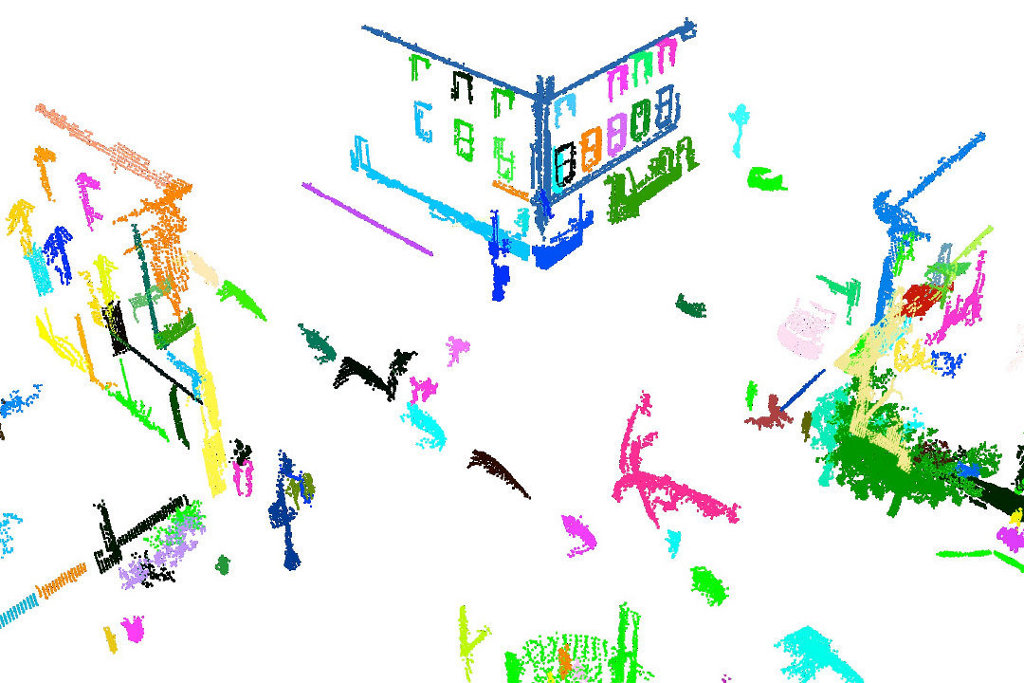# Difference of Normals Based Segmentation

In this tutorial we will learn how to use Difference of Normals features, implemented in the pcl::DifferenceOfNormalsEstimation class, for scale-based segmentation of unorganized point clouds.

This algorithm performs a scale based segmentation of the given input point cloud, finding points that belong within the scale parameters given.Overview of the pipeline in DoN segmentation.

## Theoretical Primer

The Difference of Normals (DoN) provides a computationally efficient, multi-scale approach to processing large unorganized 3D point clouds. The idea is very simple in concept, and yet surprisingly effective in the segmentation of scenes with a wide variation of scale. For each pointin a pointcloud, two unit point normalsare estimated with different radii,. The normalized (vector) difference of these point normals defines the operator.

Formally the Difference of Normals operator is defined,where,, andis the surface normal estimate at point, given the support radius. Notice, the response of the operator is a normalized vector field, and is thus orientable (the resulting direction is a key feature), however the operator’s norm often provides an easier quantity to work with, and is always in the range.Illustration of the effect of support radius on estimated surface normals for a point cloud.

The primary motivation behind DoN is the observation that surface normals estimated at any given radius reflect the underlying geometry of the surface at the scale of the support radius. Although there are many different methods of estimating the surface normals, normals are always estimated with a support radius (or via a fixed number of neighbours). This support radius determines the scale in the surface structure which the normal represents.

The above diagram illustrates this effect in 1D. Normals,, and tangents,, estimated with a small support radiusare affected by small-scale surface structure (and similarly by noise). On the other hand, normals and tangent planes estimated with a large support radius $r_l$ are less affected by small-scale structure, and represent the geometry of larger scale surface structures. In fact a similar set of features is seen in the DoN feature vectors for real-world street curbs in a LiDAR image shown below.Closeup of the DoN feature vectors calculated for a LiDAR pointcloud of a street curb.

For more comprehensive information, please refer to the article [DON2012].

## Using Difference of Normals for Segmentation

For segmentation we simply perform the following:

1. Estimate the normals for every point using a large support radius of2. Estimate the normals for every point using the small support radius of3. For every point the normalized difference of normals for every point, as defined above.
4. Filter the resulting vector field to isolate points belonging to the scale/region of interest.

## The Data Set

For this tutorial we suggest the use of publicly available (creative commons licensed) urban LiDAR data from the [KITTI] project. This data is collected from a Velodyne LiDAR scanner mounted on a car, for the purpose of evaluating self-driving cars. To convert the data set to PCL compatible point clouds please see [KITTIPCL]. Examples and an example data set will be posted here in future as part of the tutorial.

## The Code

Next what you need to do is to create a file don_segmentation.cpp in any editor you prefer and copy the following code inside of it:

  1 2 3 4 5 6 7 8 9 10 11 12 13 14 15 16 17 18 19 20 21 22 23 24 25 26 27 28 29 30 31 32 33 34 35 36 37 38 39 40 41 42 43 44 45 46 47 48 49 50 51 52 53 54 55 56 57 58 59 60 61 62 63 64 65 66 67 68 69 70 71 72 73 74 75 76 77 78 79 80 81 82 83 84 85 86 87 88 89 90 91 92 93 94 95 96 97 98 99 100 101 102 103 104 105 106 107 108 109 110 111 112 113 114 115 116 117 118 119 120 121 122 123 124 125 126 127 128 129 130 131 132 133 134 135 136 137 138 139 140 141 142 143 144 145 146 147 148 149 150 151 152 153 154 155 156 157 158 159 160 161 162 163 164 165 166 167 168 169 170 171 172 173 174 175 176 177 178 179 180 181 182 183 184 185 186 187 188 189 /** * @file don_segmentation.cpp * Difference of Normals Example for PCL Segmentation Tutorials. * * @author Yani Ioannou * @date 2012-09-24 */ #include #include #include #include #include #include #include #include #include using namespace pcl; int main (int argc, char *argv[]) { ///The smallest scale to use in the DoN filter. double scale1; ///The largest scale to use in the DoN filter. double scale2; ///The minimum DoN magnitude to threshold by double threshold; ///segment scene into clusters with given distance tolerance using euclidean clustering double segradius; if (argc < 6) { std::cerr << "usage: " << argv << " inputfile smallscale largescale threshold segradius" << std::endl; exit (EXIT_FAILURE); } /// the file to read from. std::string infile = argv; /// small scale std::istringstream (argv) >> scale1; /// large scale std::istringstream (argv) >> scale2; std::istringstream (argv) >> threshold; // threshold for DoN magnitude std::istringstream (argv) >> segradius; // threshold for radius segmentation // Load cloud in blob format pcl::PCLPointCloud2 blob; pcl::io::loadPCDFile (infile.c_str (), blob); pcl::PointCloud::Ptr cloud (new pcl::PointCloud); pcl::fromPCLPointCloud2 (blob, *cloud); // Create a search tree, use KDTreee for non-organized data. pcl::search::Search::Ptr tree; if (cloud->isOrganized ()) { tree.reset (new pcl::search::OrganizedNeighbor ()); } else { tree.reset (new pcl::search::KdTree (false)); } // Set the input pointcloud for the search tree tree->setInputCloud (cloud); if (scale1 >= scale2) { std::cerr << "Error: Large scale must be > small scale!" << std::endl; exit (EXIT_FAILURE); } // Compute normals using both small and large scales at each point pcl::NormalEstimationOMP ne; ne.setInputCloud (cloud); ne.setSearchMethod (tree); /** * NOTE: setting viewpoint is very important, so that we can ensure * normals are all pointed in the same direction! */ ne.setViewPoint (std::numeric_limits::max (), std::numeric_limits::max (), std::numeric_limits::max ()); // calculate normals with the small scale std::cout << "Calculating normals for scale..." << scale1 << std::endl; pcl::PointCloud::Ptr normals_small_scale (new pcl::PointCloud); ne.setRadiusSearch (scale1); ne.compute (*normals_small_scale); // calculate normals with the large scale std::cout << "Calculating normals for scale..." << scale2 << std::endl; pcl::PointCloud::Ptr normals_large_scale (new pcl::PointCloud); ne.setRadiusSearch (scale2); ne.compute (*normals_large_scale); // Create output cloud for DoN results PointCloud::Ptr doncloud (new pcl::PointCloud); copyPointCloud (*cloud, *doncloud); std::cout << "Calculating DoN... " << std::endl; // Create DoN operator pcl::DifferenceOfNormalsEstimation don; don.setInputCloud (cloud); don.setNormalScaleLarge (normals_large_scale); don.setNormalScaleSmall (normals_small_scale); if (!don.initCompute ()) { std::cerr << "Error: Could not initialize DoN feature operator" << std::endl; exit (EXIT_FAILURE); } // Compute DoN don.computeFeature (*doncloud); // Save DoN features pcl::PCDWriter writer; writer.write ("don.pcd", *doncloud, false); // Filter by magnitude std::cout << "Filtering out DoN mag <= " << threshold << "..." << std::endl; // Build the condition for filtering pcl::ConditionOr::Ptr range_cond ( new pcl::ConditionOr () ); range_cond->addComparison (pcl::FieldComparison::ConstPtr ( new pcl::FieldComparison ("curvature", pcl::ComparisonOps::GT, threshold)) ); // Build the filter pcl::ConditionalRemoval condrem; condrem.setCondition (range_cond); condrem.setInputCloud (doncloud); pcl::PointCloud::Ptr doncloud_filtered (new pcl::PointCloud); // Apply filter condrem.filter (*doncloud_filtered); doncloud = doncloud_filtered; // Save filtered output std::cout << "Filtered Pointcloud: " << doncloud->size () << " data points." << std::endl; writer.write ("don_filtered.pcd", *doncloud, false); // Filter by magnitude std::cout << "Clustering using EuclideanClusterExtraction with tolerance <= " << segradius << "..." << std::endl; pcl::search::KdTree::Ptr segtree (new pcl::search::KdTree); segtree->setInputCloud (doncloud); std::vector cluster_indices; pcl::EuclideanClusterExtraction ec; ec.setClusterTolerance (segradius); ec.setMinClusterSize (50); ec.setMaxClusterSize (100000); ec.setSearchMethod (segtree); ec.setInputCloud (doncloud); ec.extract (cluster_indices); int j = 0; for (std::vector::const_iterator it = cluster_indices.begin (); it != cluster_indices.end (); ++it, j++) { pcl::PointCloud::Ptr cloud_cluster_don (new pcl::PointCloud); for (std::vector::const_iterator pit = it->indices.begin (); pit != it->indices.end (); ++pit) { cloud_cluster_don->points.push_back ((*doncloud)[*pit]); } cloud_cluster_don->width = cloud_cluster_don->size (); cloud_cluster_don->height = 1; cloud_cluster_don->is_dense = true; //Save cluster std::cout << "PointCloud representing the Cluster: " << cloud_cluster_don->size () << " data points." << std::endl; std::stringstream ss; ss << "don_cluster_" << j << ".pcd"; writer.write (ss.str (), *cloud_cluster_don, false); } } 

### Compiling and running the program

  1 2 3 4 5 6 7 8 9 10 11 12 cmake_minimum_required(VERSION 2.8 FATAL_ERROR) project(don_segmentation) find_package(PCL 1.7 REQUIRED) include_directories(${PCL_INCLUDE_DIRS}) link_directories(${PCL_LIBRARY_DIRS}) add_definitions(${PCL_DEFINITIONS}) add_executable (don_segmentation don_segmentation.cpp) target_link_libraries (don_segmentation${PCL_LIBRARIES}) 

Create a build directory, and build the executable:

$mkdir build$ cd build
$cmake ..$ make


After you have made the executable, you can run it. Simply run:

\$ ./don_segmentation <inputfile> <smallscale> <largescale> <threshold> <segradius>


## The Explanation

  pcl::search::Search<PointXYZRGB>::Ptr tree;
if (cloud->isOrganized ())
{
tree.reset (new pcl::search::OrganizedNeighbor<PointXYZRGB> ());
}
else
{
tree.reset (new pcl::search::KdTree<PointXYZRGB> (false));
}

// Set the input pointcloud for the search tree
tree->setInputCloud (cloud);


We will skip the code for loading files and parsing command line arguments, and go straight to the first major PCL calls. For our later calls to calculate normals, we need to create a search tree. For organized data (i.e. a depth image), a much faster search tree is the OrganizedNeighbor search tree. For unorganized data, i.e. LiDAR scans, a KDTree is a good option.

  pcl::NormalEstimationOMP<PointXYZRGB, PointNormal> ne;
ne.setInputCloud (cloud);
ne.setSearchMethod (tree);

/**
* NOTE: setting viewpoint is very important, so that we can ensure
* normals are all pointed in the same direction!
*/
ne.setViewPoint (std::numeric_limits<float>::max (), std::numeric_limits<float>::max (), std::numeric_limits<float>::max ());

// calculate normals with the small scale


This is perhaps the most important section of code, estimating the normals. This is also the bottleneck computationally, and so we will use the pcl::NormalEstimationOMP class which makes use of OpenMP to use many threads to calculate the normal using the multiple cores found in most modern processors. We could also use the standard single-threaded class pcl::NormalEstimation, or even the GPU accelerated class pcl::gpu::NormalEstimation. Whatever class we use, it is important to set an arbitrary viewpoint to be used across all the normal calculations - this ensures that normals estimated at different scales share a consistent orientation.

Note

For information and examples on estimating normals, normal ambiguity, and the different normal estimation methods in PCL, please read the Estimating Surface Normals in a PointCloud tutorial.

  std::cout << "Calculating normals for scale..." << scale1 << std::endl;
pcl::PointCloud<PointNormal>::Ptr normals_small_scale (new pcl::PointCloud<PointNormal>);

ne.compute (*normals_small_scale);

// calculate normals with the large scale
std::cout << "Calculating normals for scale..." << scale2 << std::endl;
pcl::PointCloud<PointNormal>::Ptr normals_large_scale (new pcl::PointCloud<PointNormal>);

ne.compute (*normals_large_scale);


Next we calculate the normals using our normal estimation class for both the large and small radius. It is important to use the NormalEstimation.setRadiusSearch() method v.s. the NormalEstimation.setMaximumNeighbours() method or equivalent. If the normal estimate is restricted to a set number of neighbours, it may not be based on the complete surface of the given radius, and thus is not suitable for the Difference of Normals features.

Note

For large supporting radii in dense point clouds, calculating the normal would be a very computationally intensive task potentially utilizing thousands of points in the calculation, when hundreds are more than enough for an accurate estimate. A simple method to speed up the calculation is to uniformly subsample the pointcloud when doing a large radius search, see the full example code in the PCL distribution at examples/features/example_difference_of_normals.cpp for more details.

### Difference of Normals Feature Calculation

  PointCloud<PointNormal>::Ptr doncloud (new pcl::PointCloud<PointNormal>);
copyPointCloud (*cloud, *doncloud);


We can now perform the actual Difference of Normals feature calculation using our normal estimates. The Difference of Normals result is a vector field, so we initialize the point cloud to store the results in as a pcl::PointNormal point cloud, and copy the points from our input pointcloud over to it, so we have what may be regarded as an uninitialized vector field for our point cloud.

  pcl::DifferenceOfNormalsEstimation<PointXYZRGB, PointNormal, PointNormal> don;
don.setInputCloud (cloud);
don.setNormalScaleLarge (normals_large_scale);
don.setNormalScaleSmall (normals_small_scale);

if (!don.initCompute ())
{
std::cerr << "Error: Could not initialize DoN feature operator" << std::endl;
exit (EXIT_FAILURE);
}

// Compute DoN
don.computeFeature (*doncloud);


We instantiate a new pcl::DifferenceOfNormalsEstimation class to take care of calculating the Difference of Normals vector field.

The pcl::DifferenceOfNormalsEstimation class has 3 template parameters, the first corresponds to the input point cloud type, in this case pcl::PointXYZRGB, the second corresponds to the type of the normals estimated for the point cloud, in this case pcl::PointNormal, and the third corresponds to the vector field output type, in this case also pcl::PointNormal. Next we set the input point cloud and give both of the normals estimated for the point cloud, and check that the requirements for computing the features are satisfied using the pcl::DifferenceOfNormalsEstimation::initCompute() method. Finally we compute the features by calling the pcl::DifferenceOfNormalsEstimation::computeFeature() method.

Note

The pcl::DifferenceOfNormalsEstimation class expects the given point cloud and normal point clouds indices to match, i.e. the first point in the input point cloud’s normals should also be the first point in the two normal point clouds.

### Difference of Normals Based Filtering

While we now have a Difference of Normals vector field, we still have the complete point set. To begin the segmentation process, we must actually discriminate points based on their Difference of Normals vector result. There are a number of common quantities you may want to try filtering by:

Quantity PointNormal Field Description Usage Scenariofloat normal DoN vector Filtering points by relative DoN angle.float curvature DoNnorm Filtering points by scale membership, large magnitude indicates point has a strong response at then given scale parameters, float normal DoN vector x component Filtering points by orientable scale, i.e. building facades with large largeand/orand small, float normal DoN vector y component, float normal DoN vector z component

In this example we will do a simple magnitude threshold, looking for objects of a scale regardless of their orientation in the scene. To do so, we must create a conditional filter:

  pcl::ConditionOr<PointNormal>::Ptr range_cond (
new pcl::ConditionOr<PointNormal> ()
);
new pcl::FieldComparison<PointNormal> ("curvature", pcl::ComparisonOps::GT, threshold))
);
// Build the filter
pcl::ConditionalRemoval<PointNormal> condrem;
condrem.setCondition (range_cond);
condrem.setInputCloud (doncloud);

pcl::PointCloud<PointNormal>::Ptr doncloud_filtered (new pcl::PointCloud<PointNormal>);

// Apply filter
condrem.filter (*doncloud_filtered);


After we apply the filter we are left with a reduced pointcloud consisting of the points with a strong response with the given scale parameters.

Note

### Clustering the Results

  std::cout << "Clustering using EuclideanClusterExtraction with tolerance <= " << segradius << "..." << std::endl;

pcl::search::KdTree<PointNormal>::Ptr segtree (new pcl::search::KdTree<PointNormal>);
segtree->setInputCloud (doncloud);

std::vector<pcl::PointIndices> cluster_indices;
pcl::EuclideanClusterExtraction<PointNormal> ec;

ec.setMinClusterSize (50);
ec.setMaxClusterSize (100000);
ec.setSearchMethod (segtree);
ec.setInputCloud (doncloud);
ec.extract (cluster_indices);


Finally, we are usually left with a number of objects or regions with good isolation, allowing us to use a simple clustering algorithm to segment the results. In this example we used Euclidean Clustering with a threshold equal to the small radius parameter.

Note

After the segmentation the cloud viewer window will be opened and you will see something similar to those images:### References/Further Information

 [DON2012] “Difference of Normals as a Multi-Scale Operator in Unorganized Point Clouds” .

Note

@ARTICLE{2012arXiv1209.1759I, author = {{Ioannou}, Y. and {Taati}, B. and {Harrap}, R. and {Greenspan}, M.}, title = “{Difference of Normals as a Multi-Scale Operator in Unorganized Point Clouds}”, journal = {ArXiv e-prints}, archivePrefix = “arXiv”, eprint = {1209.1759}, primaryClass = “cs.CV”, keywords = {Computer Science - Computer Vision and Pattern Recognition}, year = 2012, month = sep, }

 [KITTI] “The KITTI Vision Benchmark Suite” .
 [KITTIPCL] “KITTI PCL Toolkit”